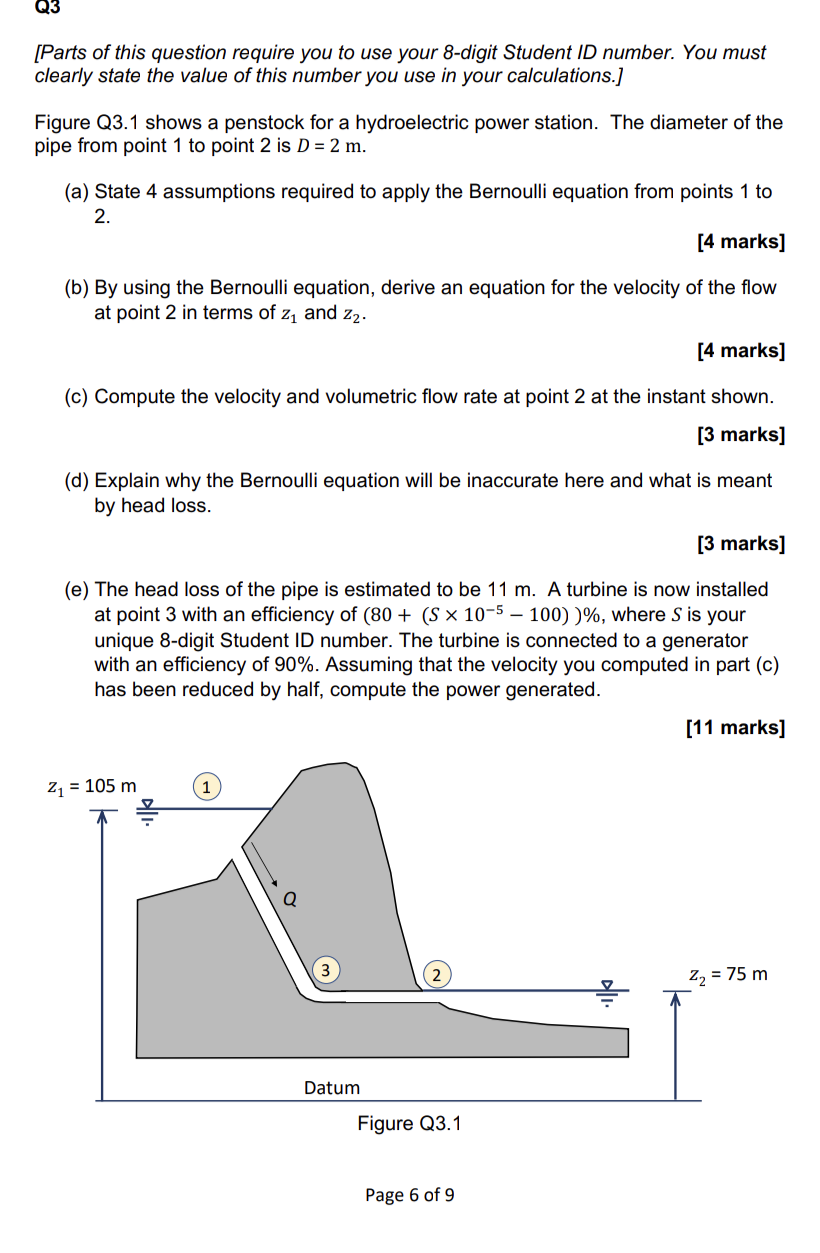# (Solved):   can someone help with this d, e please [Parts of this question require you to use your 8- ...can someone help with this d, e please

[Parts of this question require you to use your 8-digit Student ID number. You must clearly state the value of this number you use in your calculations.] Figure Q3.1 shows a penstock for a hydroelectric power station. The diameter of the pipe from point 1 to point 2 is $$D=2 \mathrm{~m}$$. (a) State 4 assumptions required to apply the Bernoulli equation from points 1 to $$2 .$$ [4 marks] (b) By using the Bernoulli equation, derive an equation for the velocity of the flow at point 2 in terms of $$z_{1}$$ and $$z_{2}$$. [4 marks] (c) Compute the velocity and volumetric flow rate at point 2 at the instant shown. [3 marks] (d) Explain why the Bernoulli equation will be inaccurate here and what is meant by head loss. [3 marks] (e) The head loss of the pipe is estimated to be $$11 \mathrm{~m}$$. A turbine is now installed at point 3 with an efficiency of $$\left(80+\left(S \times 10^{-5}-100\right)\right) \%$$, where $$S$$ is your unique 8-digit Student ID number. The turbine is connected to a generator with an efficiency of $$90 \%$$. Assuming that the velocity you computed in part (c) has been reduced by half, compute the power generated. Page 6 of 9

We have an Answer from Expert